# Professor Layton and the Unwound Future: A Weekly Puzzles Guide

## Getting Started With The First Five Puzzles

Using the Nintendo DS Wi-Fi Connection, you can download extra Professor Layton and the Unwound Future "weekly puzzles" through the game's "Bonuses" section. Starting with the game's release in September 2010, they've added one more puzzle every week until the 33rd and final puzzle was released on May 1st, 2011. For those of you that have been missing solving puzzles since finishing the game, they're a great addition, and in case you get stuck on any, what follows is a complete guide to all 33 of them. Read on for the solutions:

Weekly Puzzle #1 – Ten Cent Increments:

Goal: Determine the four stamps that can be combined in different groupings to create all of the ten cent increments from 10¢ up to the highest possible value.

Solution: The 10, 20, 40, and 80 cent stamps are the four that can be combined to solve this puzzle.

Weekly Puzzle #2 – Sporting Goods:

Goal: Determine which of the pieces of sports equipment the athlete in the puzzle would be unable to perform their best with.

Solution: All of the items shown can be used identically by left and right-handed people, with the exception of the golf club. The only one that could affect the athlete would be the golf club. Check its box to solve the puzzle.

Weekly Puzzle #3 – A Dicey Treasure Map:

Goal: Use the dice provided to indicate the location of the treasure at the far left of the squares shown.

Solution: The dice can be rotated. Rotate the 3 dice showing "6" on their face by 90 degrees and place them on the far right. In the two squares stacked vertically on each other to the left of those, place one of the dice showing "3" on the face in the top square, and rotate the other "3" 90 degrees before placing it in the bottom square. Finally, place the dice showing "1" in the far left square. This will create an arrow pointing to the space at the far left, where the treasure is hidden.

Weekly Puzzle #4 – Analog Angles:

Goal: Choose the time, of the 3 choices provided, that forms the largest angle between the two hands of an analog clock.

Solution: Keep in mind that the puzzle doesn't specify that you're looking for the largest inner angle; it simply says the largest angle. The solution is (B) 2:20, the time that creates the smallest inner angle, because its outer angle is the largest overall angle created by any of the choices.

Goal: On the chart provided, use the symbols given to mark the different types of bread eaten for every meal over the course of a week.

Solution: The first row of the chart, the morning, should read as follows from left to right: X, circle, X, circle, X, circle, X. The midday row should read: square, X, square, X, square, X, square. Finally, from left to right, the final row for night-time should read X, triangle, X, triangle, X, circle, X.

## Puzzles 6 and 7: We Hope You Like Slide Puzzles!

Weekly Puzzle #6 – Underground Escape: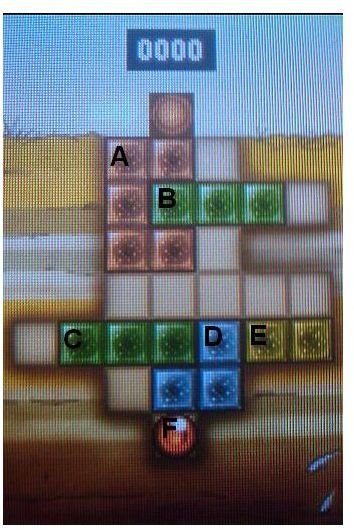Goal: Move the orange ball to the exit of the slide puzzle.

Solution: Each piece will be labeled in the image to the right, and the solution is as follows:

• First, slide the yellow block (piece E) up one square and left three squares.
• Next, slide the green block on the bottom (piece C) one square to the left.
• Slide the blue block (piece D) up one space and to the right two spaces.
• Move E right two spaces.
• Now drag the orange ball (piece F) up three squares.
• Then, move C two spaces to the right and one space down.
• Next, drag F down one square and three squares to the left.
• Move the green block at the top (piece B) to the right one space.
• Then, move the orange block (piece A) down two squares and then one square to the right.
• Finally, drag F two spaces to the right, four spaces up, one more to the right, and then one final space up to the exit.

Weekly Puzzle #7 – Packed In: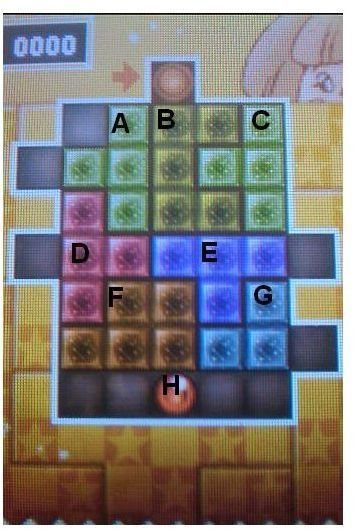Goal: Move the orange ball to the exit of the slide puzzle.

Solution: Just as in the previous puzzle, the solution is as follows, with the pieces labeled in the image to the right:

• Begin by sliding the orange ball (piece H) two spaces to the right.
• Now, slide the orange piece in the lower left corner (piece F) down one square.
• Then, drag the red block (piece D) down one square.
• Move the green piece in the top left corner (piece A) one square to the left.
• Next, move the purple block (piece E) left one space.
• Slide the blue block in the lower right corner (piece G) up one space.
• Then drag F one square to the right.
• Now, move D one space down.
• Next, slide E one space to the left.
• Drag G one space to the left.
• Move H up three squares and one square to the right.
• Then drag G right one square.
• Slide E one square to the right.
• Then, slide D up one space.
• Now move F left one space.
• Slide G down two spaces.
• Now drag E one square to the right and one square down.
• Drag the yellow block in the center (piece B) one space to the left and one space down.
• Now, move the green piece on the right (piece C) one space down and one to the left.
• Finally, move H one square left, three squares up, two squares left again, and one last square up into the exit.

## Rounding Out The First Dozen Puzzles: Puzzles 8-12

Weekly Puzzle #8 – Ski-Slope Sketch:

Goal: Find the area on the sketch of a woman that was mistaken for a large nose.

Solution: If you picture the raised ski goggles as eyes, the woman's bangs resemble a large nose. Draw your circle around her bangs.

Weekly Puzzle #9 – It Works Both Ways:

Goal: Place the number tiles to spell out an equation that is still correct when turned upside down.

Solution: Every one of the number tiles provided reads identically upside down, so you just need to determine an equation that reads correctly right to left as well as left to right. The correct equation is 12 x 21 = 252, which becomes 252 = 12 x 21 when turned upside down.

Weekly Puzzle #10 – Bandage Bonanza:

Goal: Identify which bandage on the pile of bandages covering the man's face was placed first.

Solution: All you need to do is figure out which bandage is below all of the others; the first one placed will be the only one with none on top of it. The only bandage that doesn't overlap any others is the blue bandage in the top left. Tap that one to solve the puzzle and continue.

Weekly Puzzle #11 – Legs And Livestock:

Goal: Determine the number of cows the seller of the ranch has.

Solution: There are 30 animals, made up of cows and chickens, with 100 legs total. So there are 10 chickens on the ranch, with 2 legs each, for a total of 20 legs, and 20 cows, with 4 legs each, for a total of 80 legs, equaling 100 legs in total. Thus, there are 20 cows.

Weekly Puzzle #12 – Clever Calculation:

Goal: Place the red tiles into the squares to create a valid equation.

Solution: The tiles can be rotated, which is not a new trick for Professor Layton, but this puzzle is a little meaner than usual. Try rotating a tile 45 degrees and placing it in a square. Even though it doesn't fit properly, notice that the game will allow you to do this. Specifically, you need to rotate the plus sign 45 degrees to create a multiplication sign. The final equation should read as follows: 2 x 9 – 6 = 12.

## Photos, Gems, and Academia: Puzzles 13-17

Weekly Puzzle #13 – Souvenir Photo:

Goal: Using the clues given, determine the identity of the alien in question.

Solution: The correct answer is A: Panpolo.

Weekly Puzzle #14 – Carpet Pile:

Goal: Determine which of the carpets in the pile is on the very bottom.

Solution: Like the bandage puzzle earlier, this is simply a matter of determining which carpet isn't on top of any of the others. Follow the patterns and shapes you can see, and you'll find that the purple carpet in the top right corner is beneath all the others. Tap to select it and solve the puzzle.

Weekly Puzzle #15 – The Crown Jewels:

Goal: Find the fewest possible number of gems on the king's crown, given the clues.

Solution: To fulfill the conditions for every clue given, there have to be at least 11 gems on the crown. 11 is the solution.

Weekly Puzzle #16 – Crazy Bird:

Goal: Work out the number of each color pebble that the bird in the puzzle has collected.

Solution: With the information you're given, the only possible solution is for there to be 1 white pebble, 2 black, 4 red, and 3 blue.

Weekly Puzzle #17 – Academic Arithmetic:

Goal: Calculate the first year the school's population will exceed 400 students with the amount of new students the school admits per year.

Solution: The school increases the number of new students by ten every year, but because it's a three-year school, starting with year four one class will graduate and leave the school every year. Using this information, you can determine that by the 8th year, the school will have a population of 420 students. The solution is 8.

## Puzzles 18-21: Oh Great, Another Slide Puzzle

Weekly Puzzle #18 – Blocked In: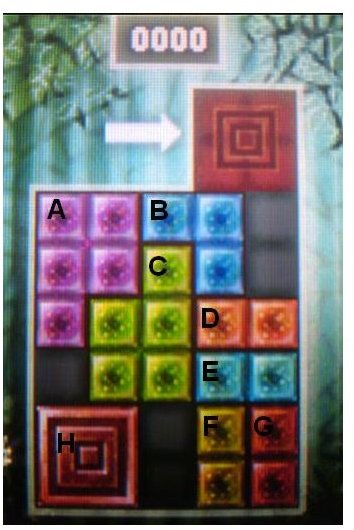Goal: Get the big red block from the bottom to the exit at the top.

Solution: The solution is shown below. Pieces are labeled in the image to the right.

• Begin by sliding the blue block in the top right corner (piece B) right one space and up two.
• Now, slide the orange block below it (piece D) up two squares and left one square.
• Next, slide the blue block below that (piece E) up two squares.
• Slide the yellow block in the bottom right corner (piece F) up two squares.
• Now move the orange block next to it (piece G) up two squares as well.
• Move the big red square (piece H) three spaces to the right.
• Then, drag the green block (piece C) down two spaces and left one space.
• Slide F left one space and down two spaces.
• Then slide E left one space and down two spaces as well.
• Drag G up two spaces.
• Then, move D down 2 squares and one square to the right.
• Slide the purple block in the top left corner (piece A) two squares to the right.
• Next, move piece C 3 spaces up.
• Drag E left two squares and down 2 squares.
• Now, move D down one space, left 3 spaces, and down one more space.
• Then, drag H up two spaces.
• Next, slide F two squares to the right.
• Then, drag D two spaces to the right.
• Now move E two spaces to the right as well.
• Next, slide C down 3 spaces.
• Now drag A two spaces to the left and one space down.
• Drag G left two spaces and down two spaces.
• Next, slide B down two squares and two squares to the left.
• Finally, move H up four squares, into the exit.

Weekly Puzzle #19 – Stained Glass:

Goal: Reassemble the scattered stained glass art to determine the source of inspiration.

Solution: Once it's been put back together, the stained glass will spell out "Layton." The inspiration was B: A person.

Weekly Puzzle #20 – Gelatinous Dessert:

Goal: Choose which of the four shapes would have been created from the dessert mold shown.

Solution: The shape that comes out of the mold will be an exact reverse image of the mold itself. The only shape that satisfies this condition is D, so that is your solution.

Weekly Puzzle #21 – Spades and Clubs:

Goal: Calculate how many cards in the pile on the right will be black cards (spades and clubs) from what you know about the other two piles.

Solution: There are 52 cards in a deck, and from the size of the first two piles, there are 17 remaining for the third pile. There are 26 red cards and 26 black cards in a deck of cards, and from the number of red cards in each of the other 2 piles, there are 16 red cards in the third pile, meaning only 1 is black. The solution is 1.

## The Slide Puzzles Just Keep On Coming: Puzzles 22-24

Weekly Puzzle #22 – Ten Candles:

Goal: Determine how many candles will be lit after 25 minutes with two people lighting candles and blowing them out at the given intervals.

Solution: There will be seven candles lit after 25 minutes.

Weekly Puzzle #23 – Books on a Table:

Goal: Identify which angle of perspective the books on the table might remind someone of Professor Layton from.

Solution: Looking at the books from angle D, the books resemble a top hat. Choose D to solve this puzzle.

Weekly Puzzle #24 – Rearranged Names:

Goal: Re-arrange the tiles to spell out the names "Layton" and "Luke"

Solution: The real trick here is that the letter L appears twice. Since the two names are color-coded, it seems that the two tiles would not be interchangeable, but the puzzle says to line up the tiles with the matching letters, and says nothing about color. The only way to solve this is to spell the names by flipping the colors for each "L" tile. The solution is as follows:

• First, slide the T tile to the right.
• Then, slide the O tile to the right as well.
• Next, slide the Top Hat tile down.
• Now, slide the A tile right.
• Then move the K tile to the right as well.
• Slide the pink L tile to the right.
• Next, slide the blue L tile up.
• Now move the Y tile to the left.
• Then move the U tile to the left as well.
• Drag the K tile down.
• Next, move the pink L tile to the right.
• Move the U tile up.
• Slide the Y tile to the right.
• Then, move the blue L tile down.
• Move the U tile left.
• Then, move the pink L tile left as well.
• Now move the A tile left.
• Next, drag the E tile to the left.
• Slide the O tile up.
• Then, move the T tile to the left.
• Now drag the N tile down.
• Next slide the O tile to the right.
• Then slide the T tile up.
• Now move the Top Hat tile to the right.
• Slide the K tile to the right.
• Next, slide the Y tile to the right as well.
• Now move the pink L tile down.
• Move the U tile to the right.
• Then slide the blue L tile up.
• Now drag the pink L tile to the left.
• Move the U tile down.
• Next, drag the A tile to the left.
• Then slide the Y tile up.
• Now, slide the K tile to the left.
• Next move the E tile down.
• Slide the T tile left.
• Slide the O tile left as well.
• Finally, slide the N tile up to finish spelling the correct names.

## At Least This Slide Puzzle Is The Last One: Puzzles 25-28

Weekly Puzzle #25 – The Messed-Up Map:

Goal: Select two segments of the map that be removed to create a map that will connect each letter at the top to its matching letter at the bottom.

Solution: Mark the horizontal line in the center of the left-most column and the horizontal line in the center of the right-most column for removal to solve this puzzle.

Weekly Puzzle #26 – The Top-Hat Society: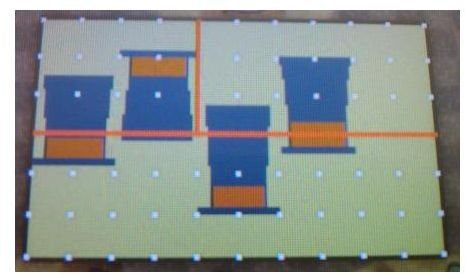Goal: Using two straight lines, cut the signboard into three pieces that, when re-arranged, can create a sign with five top hats instead of four.

Solution: Draw your lines as shown here:

Weekly Puzzle #27 – A Trio of Hearts:

Goal: Choose the three cards between the Ace and the 10 of hearts that add up to 12 when counting the actual hearts shown on the faces of the cards.

Solution: Don't forget that playing cards have hearts in the top left and bottom right corners, in addition to the hearts in the center. The cards that will add up to 12 hearts are the Ace, 2, and the 3.

Weekly Puzzle #28 – Cave Blockade: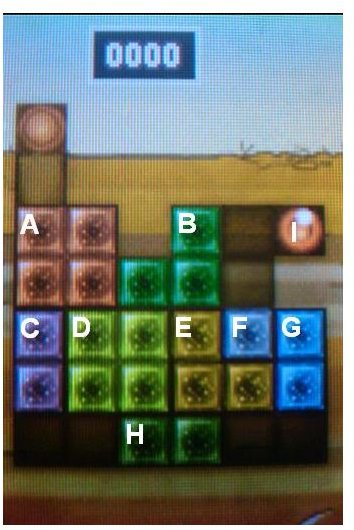Goal: Move the orange ball from its starting place on the right side to the exit on the left.

Solution: As with all slide puzzles, the solution is provided one step at a time, and the pieces in the puzzle are labeled in the image to the right.

• Begin by sliding the green block at the very bottom (piece H) two squares to the right.
• Next, slide the purple block on the left (piece C) down one space.
• Move the large yellow square next to it (piece D) down one space as well.
• Then, drag the green block in the top right corner (piece B) one square to the right.
• Now, move the big orange square in the top left corner (piece A) down one space and right one space.
• Move C up five spaces.
• Then slide A left one space and up one space.
• Now drag D up one space and left one space.
• Next, slide H left four spaces.
• Slide the yellow L-shaped block (piece E) down one space and right one space.
• Move D right two spaces and down one space.
• Slide the single-square block (piece F) left three squares and down one.
• Slide B down one space.
• Now, move the orange ball (piece I) left two squares, down one square, left another square, down another square, then left two more squares, and down one more.
• Then, move piece A down one square and right one square.
• Next slide I up 3 squares and right one square.
• Slide C down 4 spaces.
• Finally, move I left one space and up two spaces, into the exit.

## Puzzles 29-33: The Final Four!

Weekly Puzzle #29 – Phone Booths:

Goal: Of the four phone booths in the photo, choose the one that can't be used.

Solution: If you examine the shadows from the sunlight in the photo, you'll find that booth D, to appear the way it does, has to be a reflection of booth C and not an actual phone booth. The solution is D, which can't be used because it isn't really there.

Weekly Puzzle #30 – Double Vision:

Goal: Check the two letters that have identical surface areas.

Solution: If you place two of the Cs shown. next to each other with one backwards, you'll form a letter H in the center. If you do the same thing with the Es, you'll form a letter I in the center of them that is the exact same size and shape as the H, simply rotated 90 degrees. For this to be possible, E and C have to have exactly the same surface area.

Weekly Puzzle #31 – Massive Gemstones:

Goal: Calculate the fewest number of times you'd need to use the scales to determine whether the diamond or the circle is the heavier gem.

Solution: If you place the two boxes with circles on the left, and the two other boxes on the right, you've got one square and one triangle on each side, which will cancel out, leaving a simple weighing of two circles on one side and two diamonds on the other side to determine which of the two is heaviest. This can be done using the scale only once.

Weekly Puzzle #32 – It All Adds Up:

Goal: Find the smallest number that is equal to the sum of its divisors not including the number itself.

Solution: The number six is divisible by 1, 2, 3, and 6. Remove 6 from that group because it's the original number, and you're left with 1, 2, and 3, which add up to 6. The solution is 6.

Weekly Puzzle #33 – Black & White Balls:

Goal: Determine the number of white balls in the square.

Solution: There are 36 black balls around the outside of the square, which means the square's dimensions are 10×10. That makes the inner square 8×8, which is equal to 64. There are 64 white balls in the square.

## This post is part of the series: Professor Layton and the Unwound Future Puzzle Walkthrough

Stuck on a puzzle in the most recent entry in the Professor Layton series? These articles will walk you through the solution to every single puzzle in the game, including all of the available bonuses, and all of the weekly downloadable puzzles. Read on for all the answers you’ll need.